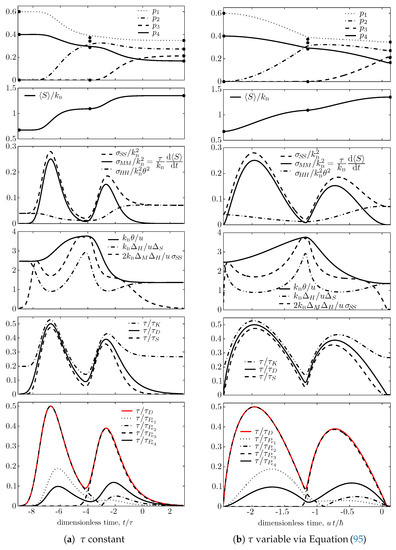Previous Article in Journal
Coexisting Attractors and Multistability in a Simple Memristive Wien-Bridge Chaotic Circuit
Previous Article in Special Issue
The Thermodynamics of Network Coding, and an Algorithmic Refinement of the Principle of Maximum Entropy

Metrics 0

## Export Article

Open AccessArticle

# Time–Energy and Time–Entropy Uncertainty Relations in Nonequilibrium Quantum Thermodynamics under Steepest-Entropy-Ascent Nonlinear Master Equations

Department of Mechanical and Industrial Engineering, Università di Brescia, via Branze 38, 25123 Brescia, Italy
Entropy 2019, 21(7), 679; https://doi.org/10.3390/e21070679
Received: 13 June 2019 / Revised: 7 July 2019 / Accepted: 8 July 2019 / Published: 11 July 2019
(This article belongs to the Special Issue Entropy Production and Its Applications: From Cosmology to Biology)
|
PDF [644 KB, uploaded 11 July 2019]
|

# Abstract

In the domain of nondissipative unitary Hamiltonian dynamics, the well-known Mandelstam–Tamm–Messiah time–energy uncertainty relation $τ F Δ H ≥ ℏ / 2$ provides a general lower bound to the characteristic time $τ F = Δ F / | d ⟨ F ⟩ / d t |$ with which the mean value of a generic quantum observable F can change with respect to the width $Δ F$ of its uncertainty distribution (square root of F fluctuations). A useful practical consequence is that in unitary dynamics the states with longer lifetimes are those with smaller energy uncertainty $Δ H$ (square root of energy fluctuations). Here we show that when unitary evolution is complemented with a steepest-entropy-ascent model of dissipation, the resulting nonlinear master equation entails that these lower bounds get modified and depend also on the entropy uncertainty $Δ S$ (square root of entropy fluctuations). For example, we obtain the time–energy-and–time–entropy uncertainty relation $( 2 τ F Δ H / ℏ ) 2 + ( τ F Δ S / k B τ ) 2 ≥ 1$ where $τ$ is a characteristic dissipation time functional that for each given state defines the strength of the nonunitary, steepest-entropy-ascent part of the assumed master equation. For purely dissipative dynamics this reduces to the time–entropy uncertainty relation $τ F Δ S ≥ k B τ$ , meaning that the nonequilibrium dissipative states with longer lifetime are those with smaller entropy uncertainty $Δ S$ . View Full-Text
FiguresFigure 1

This is an open access article distributed under the Creative Commons Attribution License which permits unrestricted use, distribution, and reproduction in any medium, provided the original work is properly cited (CC BY 4.0).MDPI and ACS Style

Beretta, G.P. Time–Energy and Time–Entropy Uncertainty Relations in Nonequilibrium Quantum Thermodynamics under Steepest-Entropy-Ascent Nonlinear Master Equations. Entropy 2019, 21, 679.

Note that from the first issue of 2016, MDPI journals use article numbers instead of page numbers. See further details here.

1